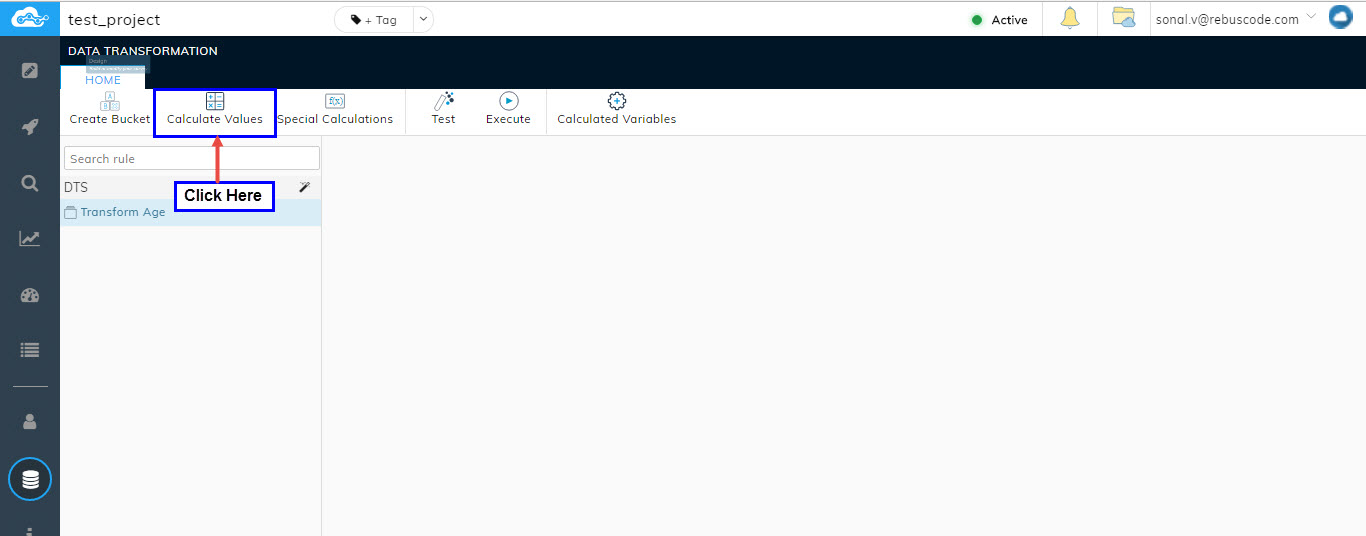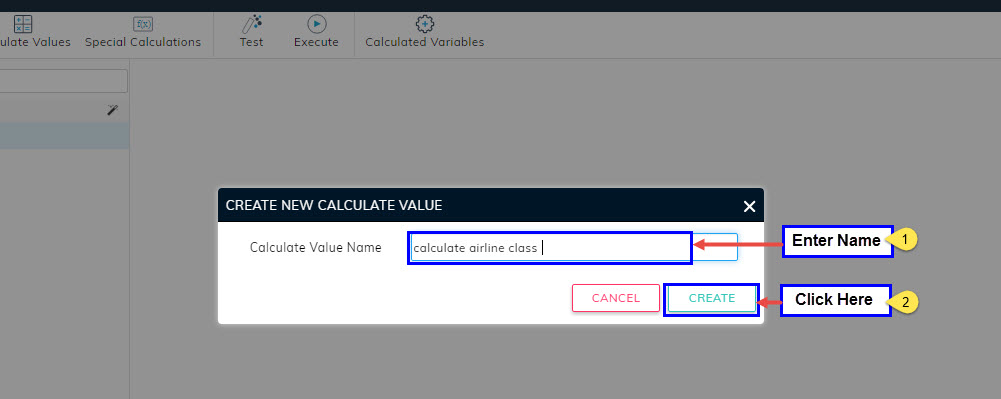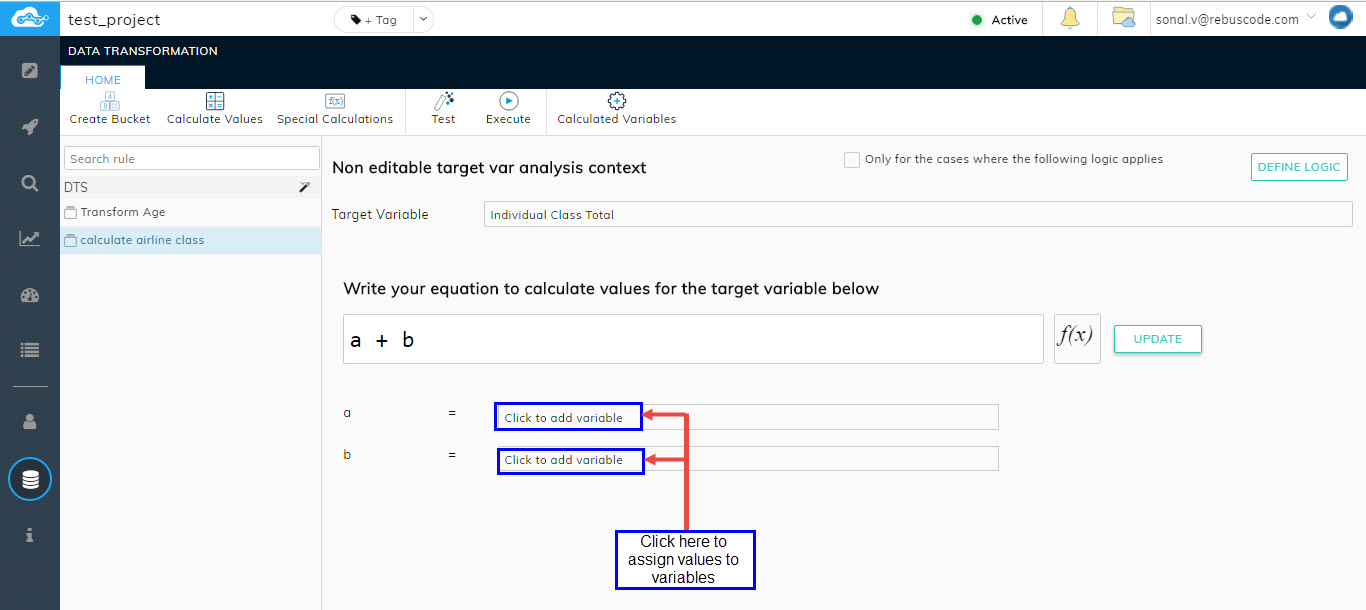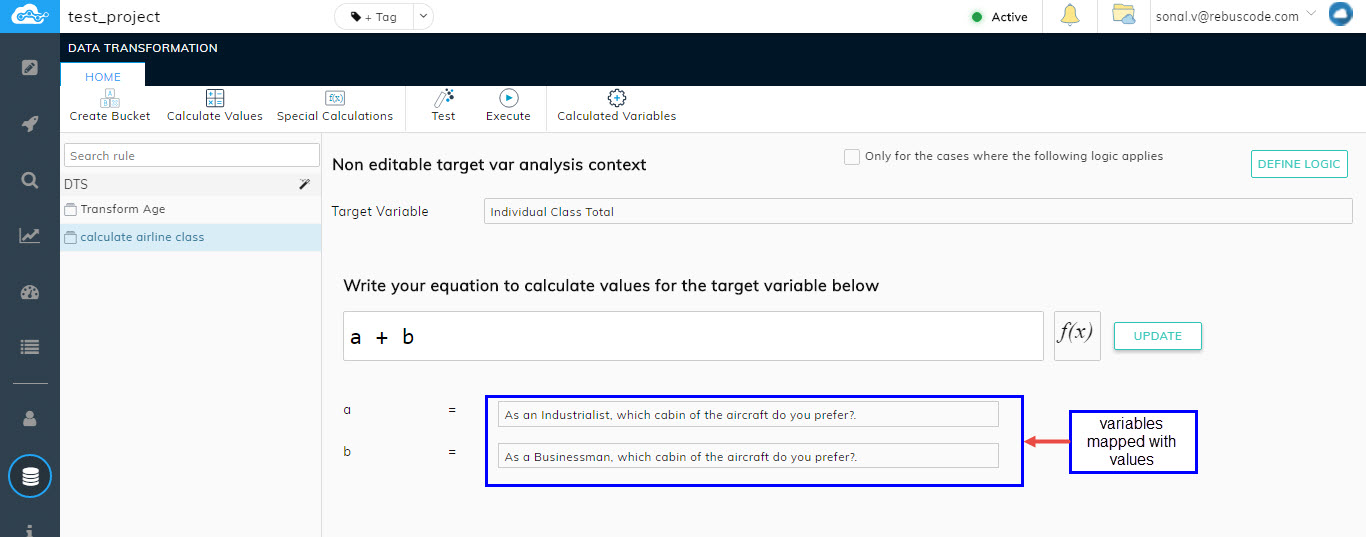The Calculate Values option allows the users to map their created calculated variable with the combined results of two or more source variables by mentioning functions of their own.

For Example: Let is consider two questions asked to the respondents belonging to two different groups.

Question-1

As an Industrialist, which cabin of the aircraft do you prefer?

• Economy
• First Class

Question-2

As a Businessman, which cabin of the aircraft do you prefer?

• Economy
• First Class

Now suppose you have created a calculated variable named "Individual Class Total" to know the total number of respondents who answered for the same class(business, economy, First class) in both the groups(Industrialists and Businessman). (Refer Section Creating Calculated Variables)

Calculated Variable

Individual Class Total

• Total Economy
• Total First ClassA pop-up will appear asking you to enter name for your calculated value. Enter name and click on Create.The calculate value will get created and will be visible in the left pane of the. All the required fields for the created calculate value will be visible in the middle of the page.Now click on Target Variable field to choose your target variable.A pop-up will appear displaying the list of all your calculated variables. Choose the required calculated variable and click on Select

In the case we are considering, let's choose the calculated variable that we have created i.e. Individual Class Total.The target variable will get added and will show up in the Target variable field.

Now, write your Function that you wish to apply in the F(x) field.

Let's assume the following:-

Question-1 = 'a'

Question-2 = 'b'

Write the function as "a + b" and click on Update.Both the values written in function will appear below with a corresponding choose variable field in front of each value. Click on "click to add variable field" against each value to select the corresponding question for your values.A pop-up will appear giving you the names of all your survey questions/variables. Choose the respective variables and click on Select.The selected variables will get added and will be visible next to your selected next to your values.In your function, you don't have to specify "=" sign because the resultant will automatically be taken as the specific Target variable which you have selected.

This completes your process of building a Calculated Value. The responses of each option belonging to both the questions selected as "a" and "b", will now automatically combine in your Calculated Variable "Individual Class Total".

Once you have specified your Data Transformation Logic, the next step is applying or Executing the logic to your data. To know how to Execute your Logic Refer Section Executing Your data Transformation Logic. Once the logic has been executed/automated, you can verify the results by going into Analyze Page.

Transforming Data on a particular Logic applied

Users can apply data transformation logic on a selected filter cut. The filter can be applied by selecting the box which says "Only for the cases where the following logic applies" and clicking on the Define Logic button.A pop-up will appear where-in you can specify your logic or condition. (Refer section Logic Builder)The data transformation logic applied on your calculated value will now work only on the data filtered out on the applied logic.